# RD Sharma Solutions for Class 9 Maths Chapter 8 Lines and Angles Exercise 8.2

RD Sharma Solutions for Class 9 is designed according to the syllabus prescribed by CBSE. In geometry, we generally come across geometrical figures consisting of angles which posses certain relations among themselves. In this exercise, students will study the relations between the angles formed between lines. Find detailed RD Sharma solutions Class 9 chapter 8 exercise 8.2 – lines and angles below.

## Download PDF of RD Sharma Solutions for Class 9 Maths Chapter 8 Lines and Angles Exercise 8.2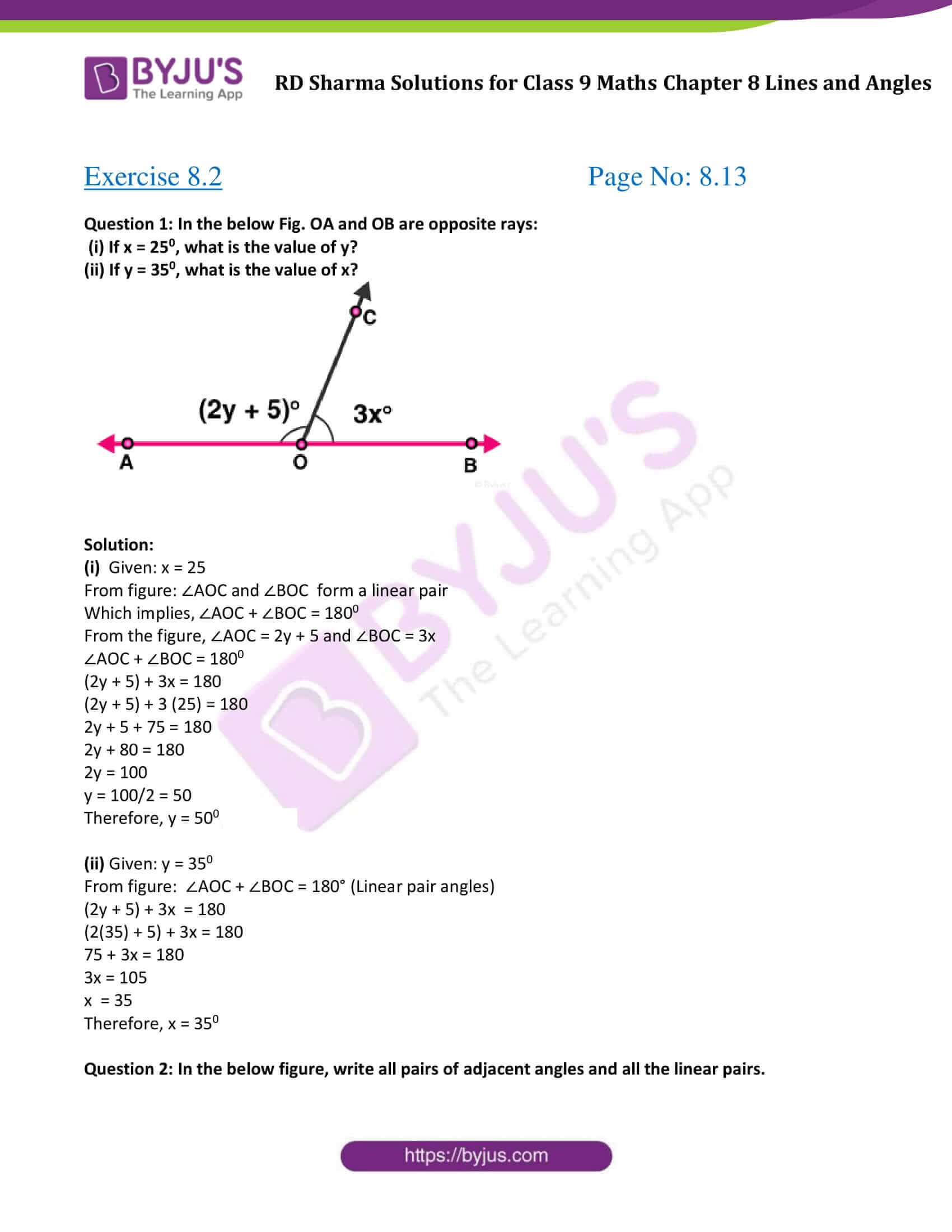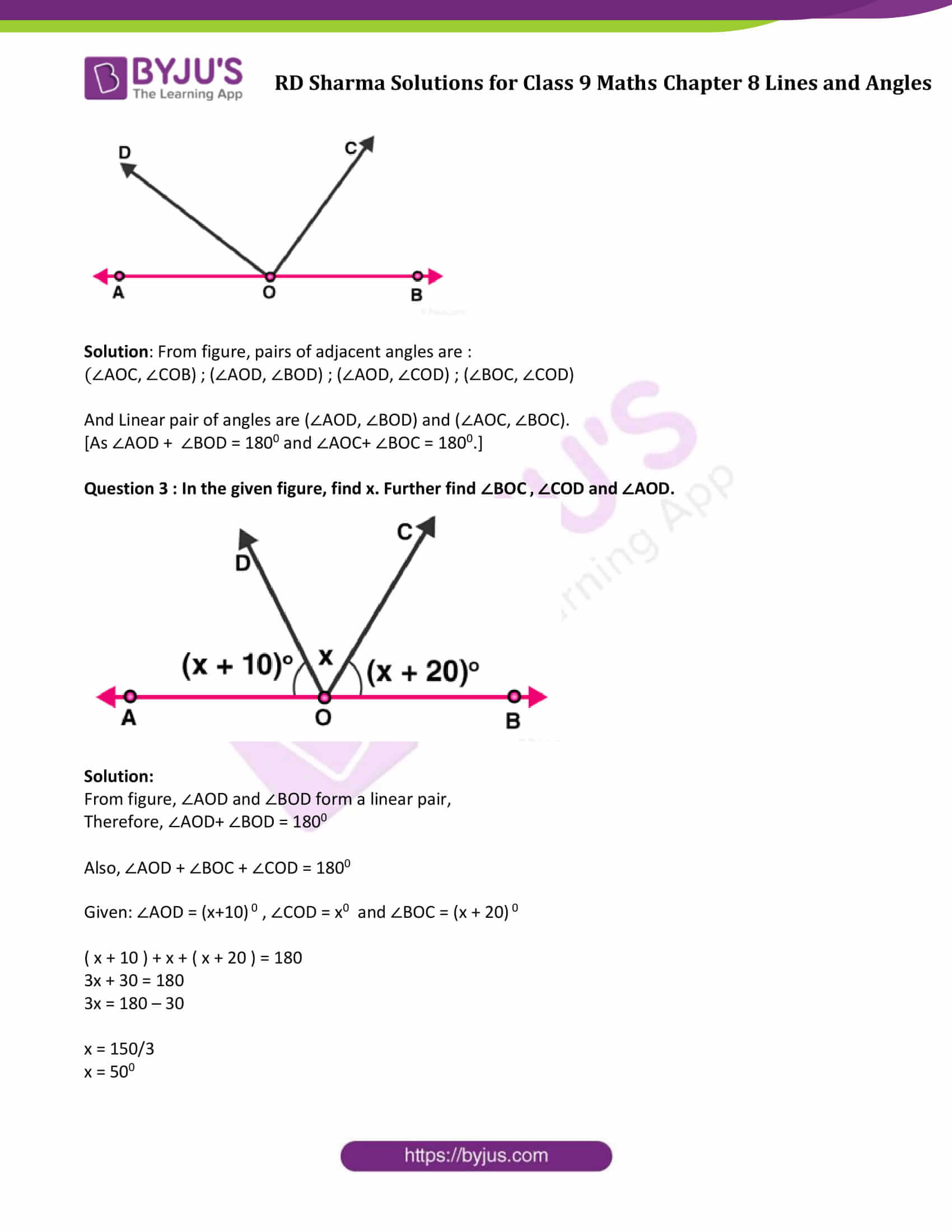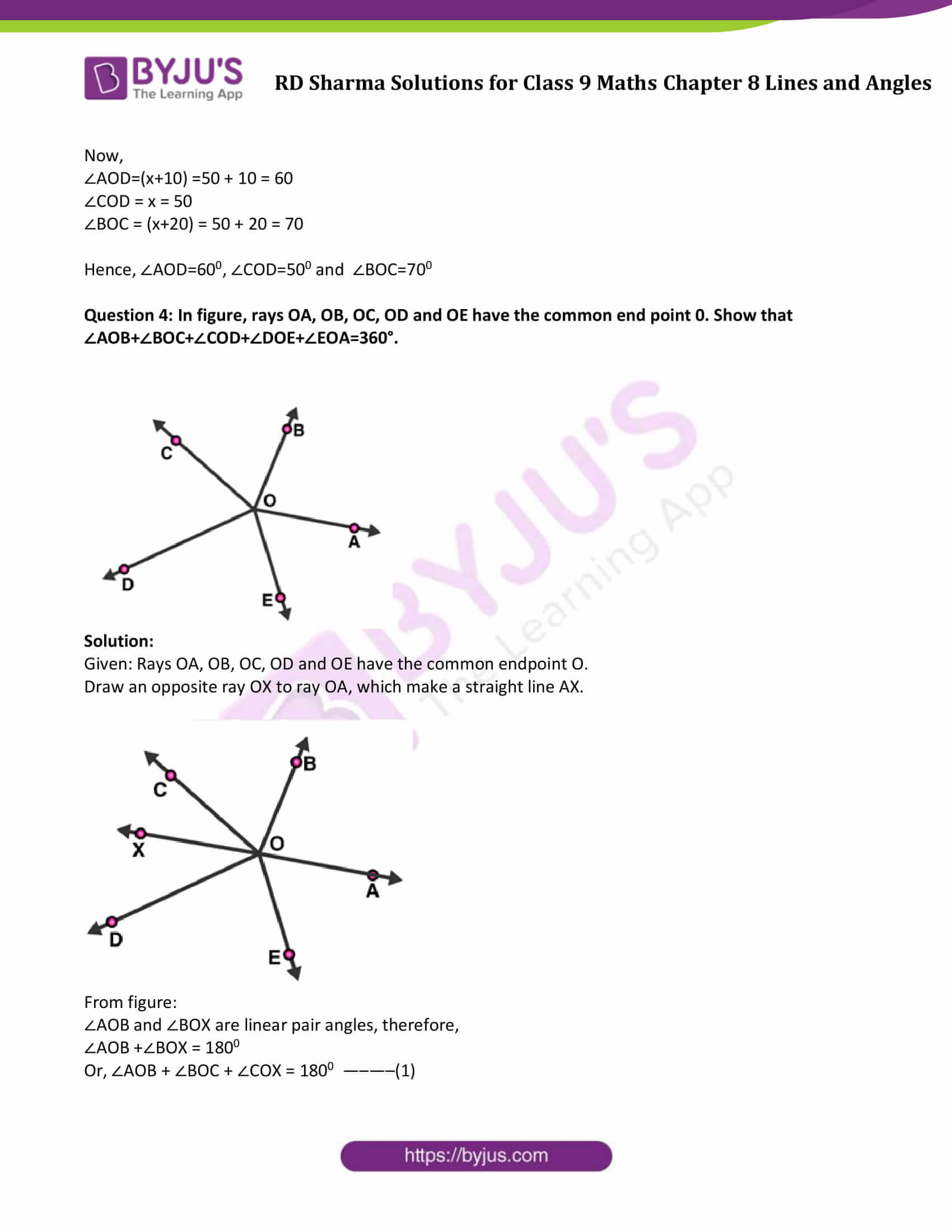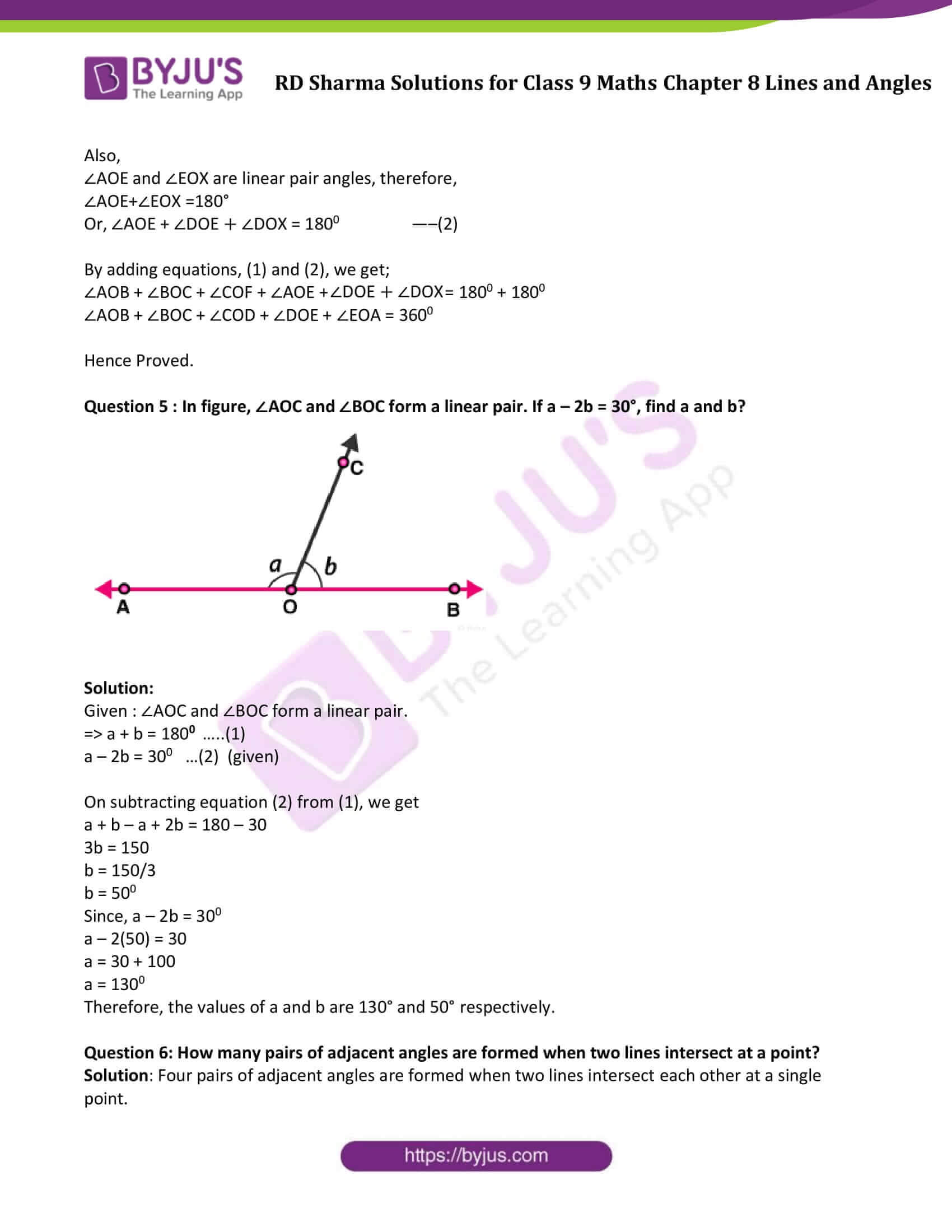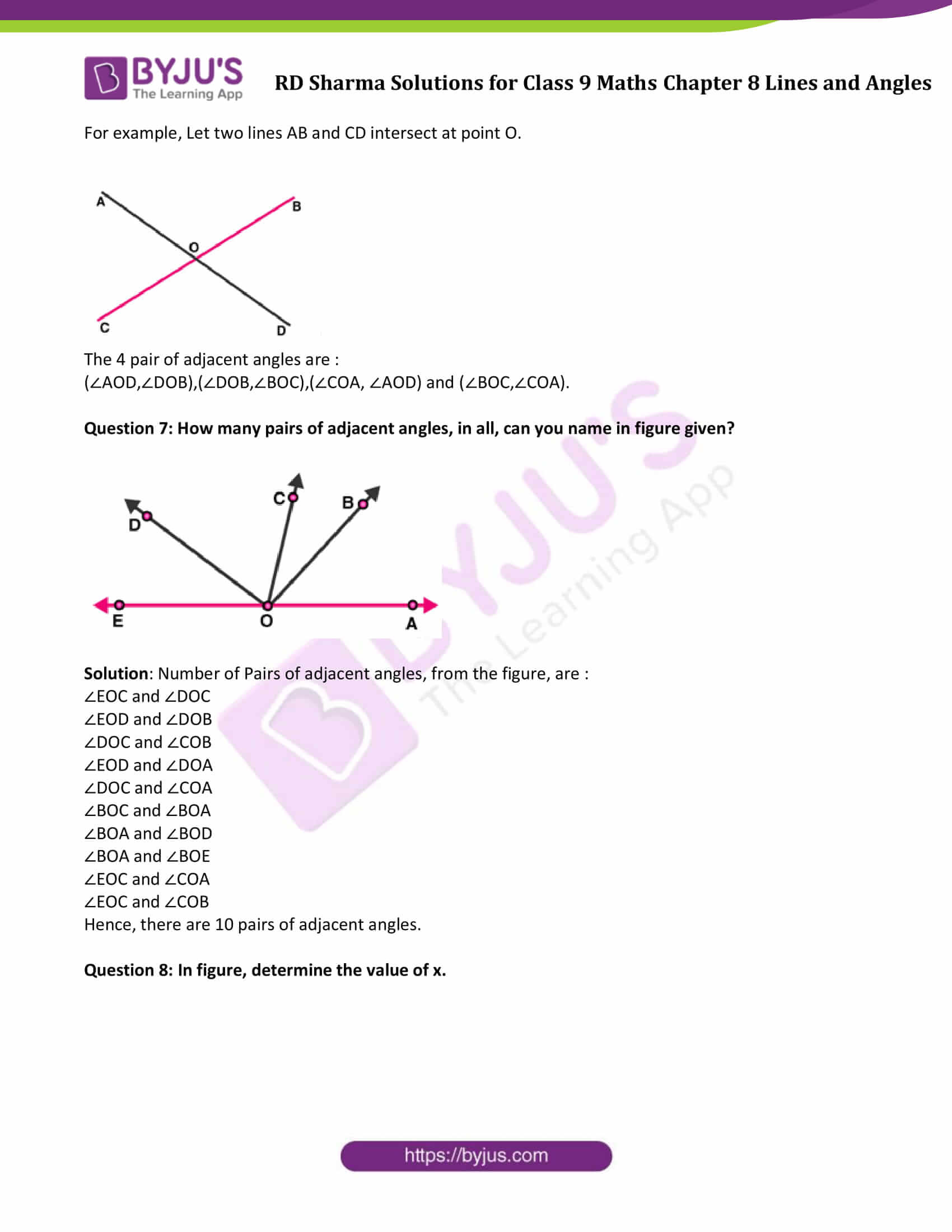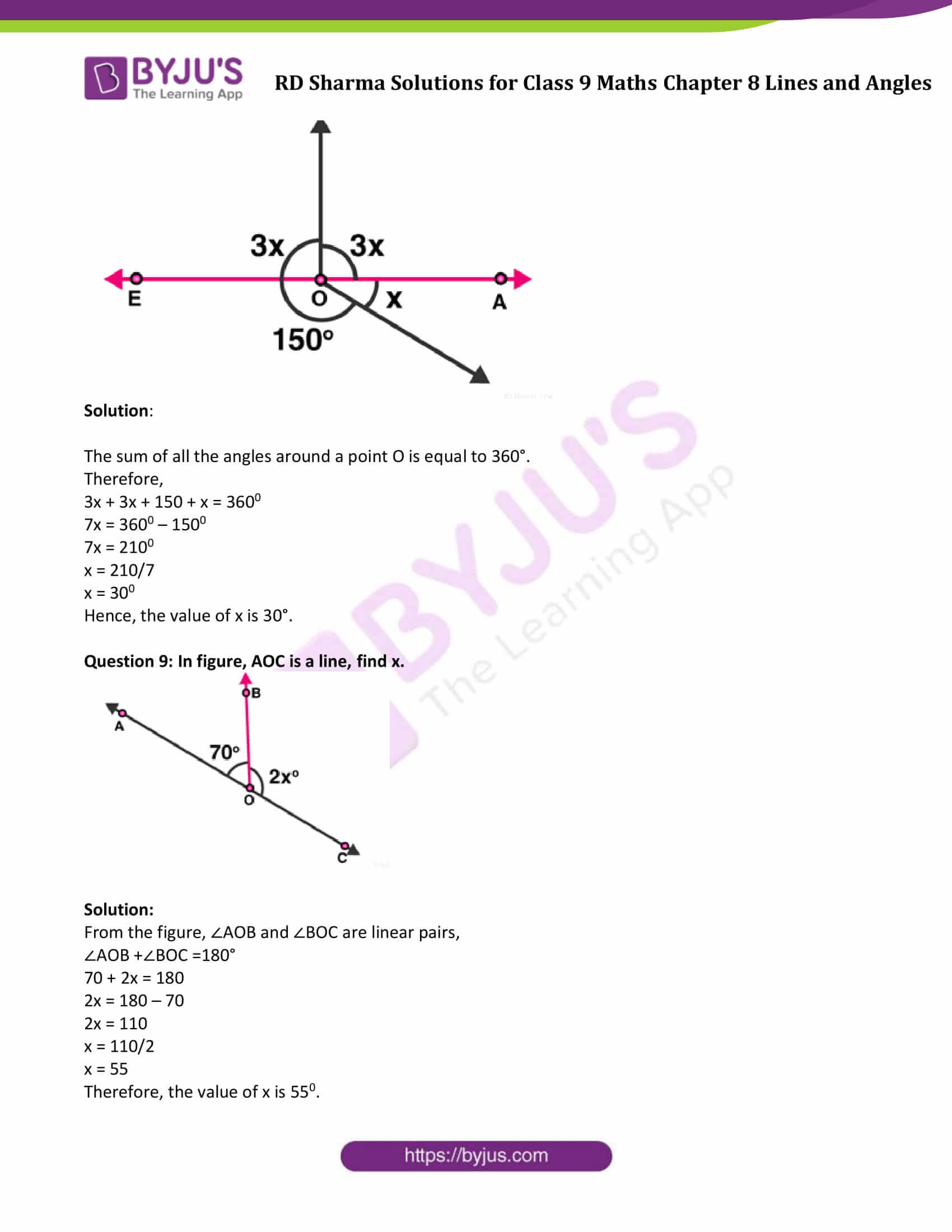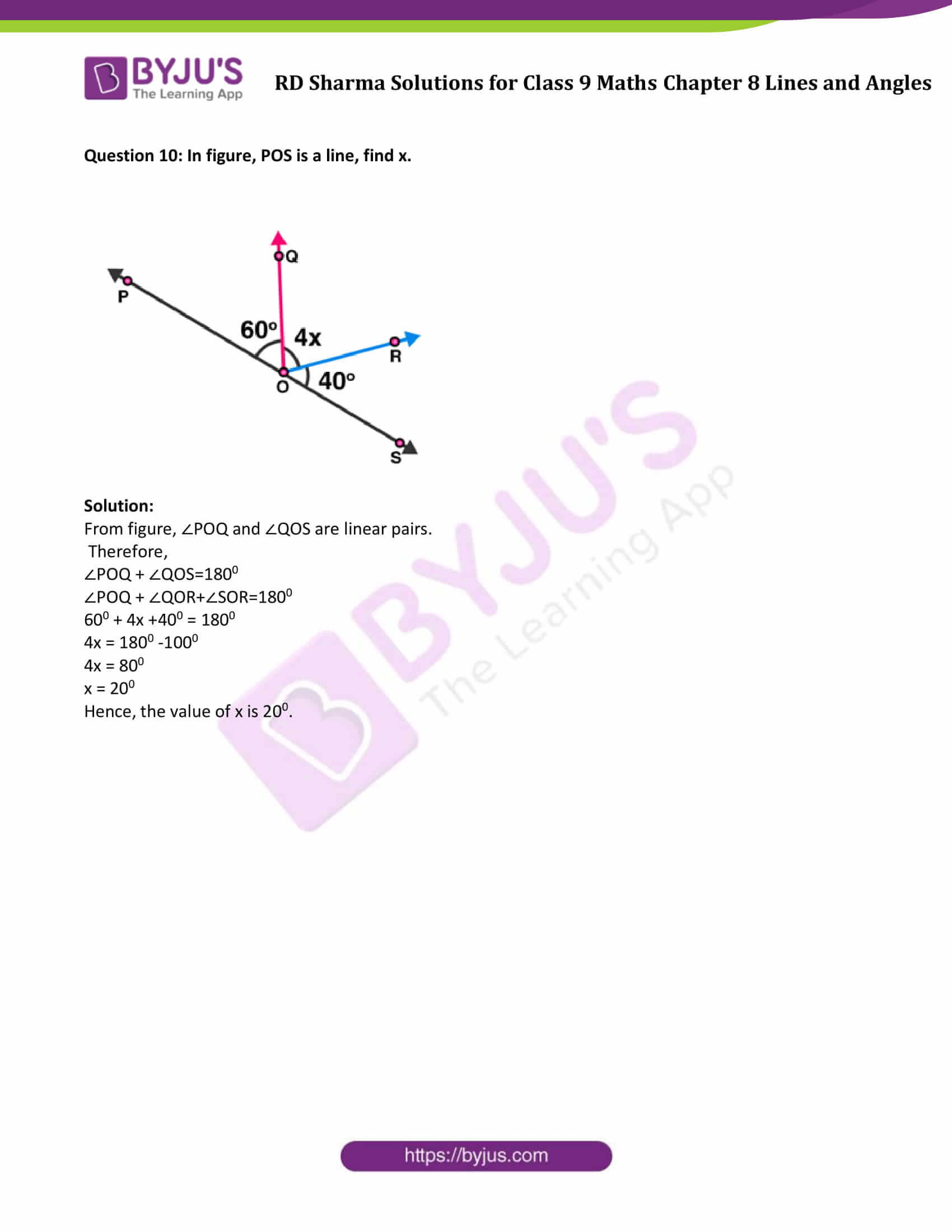### Access Answers to Maths RD Sharma Solutions for Class 9 Chapter 8 Lines and Angles Exercise 8.2 Page number 8.13

Question 1: In the below Fig. OA and OB are opposite rays:

(i) If x = 250, what is the value of y?

(ii) If y = 350, what is the value of x?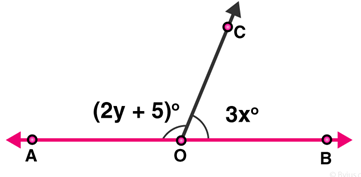Solution:

(i) Given: x = 25

From figure: ∠AOC and ∠BOC form a linear pair

Which implies, ∠AOC + ∠BOC = 1800

From the figure, ∠AOC = 2y + 5 and ∠BOC = 3x

∠AOC + ∠BOC = 1800

(2y + 5) + 3x = 180

(2y + 5) + 3 (25) = 180

2y + 5 + 75 = 180

2y + 80 = 180

2y = 100

y = 100/2 = 50

Therefore, y = 500

(ii) Given: y = 350

From figure: ∠AOC + ∠BOC = 180° (Linear pair angles)

(2y + 5) + 3x = 180

(2(35) + 5) + 3x = 180

75 + 3x = 180

3x = 105

x = 35

Therefore, x = 350

Question 2: In the below figure, write all pairs of adjacent angles and all the linear pairs.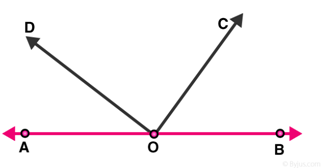Solution: From figure, pairs of adjacent angles are :

(∠AOC, ∠COB) ; (∠AOD, ∠BOD) ; (∠AOD, ∠COD) ; (∠BOC, ∠COD)

And Linear pair of angles are (∠AOD, ∠BOD) and (∠AOC, ∠BOC).

[As ∠AOD + ∠BOD = 1800 and ∠AOC+ ∠BOC = 1800.]

Question 3 : In the given figure, find x. Further find ∠BOC , ∠COD and ∠AOD.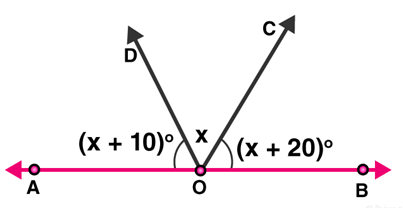Solution:

From figure, ∠AOD and ∠BOD form a linear pair,

Therefore, ∠AOD+ ∠BOD = 1800

Also, ∠AOD + ∠BOC + ∠COD = 1800

Given: ∠AOD = (x+10) 0 , ∠COD = x0 and ∠BOC = (x + 20) 0

( x + 10 ) + x + ( x + 20 ) = 180

3x + 30 = 180

3x = 180 – 30

x = 150/3

x = 500

Now,

∠AOD=(x+10) =50 + 10 = 60

∠COD = x = 50

∠BOC = (x+20) = 50 + 20 = 70

Hence, ∠AOD=600, ∠COD=500 and ∠BOC=700

Question 4: In figure, rays OA, OB, OC, OD and OE have the common end point 0. Show that ∠AOB+∠BOC+∠COD+∠DOE+∠EOA=360°.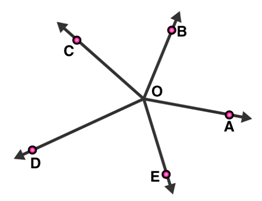Solution:

Given: Rays OA, OB, OC, OD and OE have the common endpoint O.

Draw an opposite ray OX to ray OA, which make a straight line AX.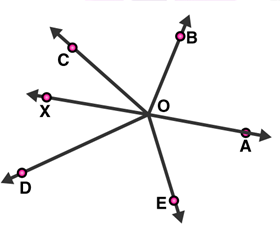From figure:

∠AOB and ∠BOX are linear pair angles, therefore,

∠AOB +∠BOX = 1800

Or, ∠AOB + ∠BOC + ∠COX = 1800 —–—–(1)

Also,

∠AOE and ∠EOX are linear pair angles, therefore,

∠AOE+∠EOX =180°

Or, ∠AOE + ∠DOE + ∠DOX = 1800 —–(2)

By adding equations, (1) and (2), we get;

∠AOB + ∠BOC + ∠COX + ∠AOE + ∠DOE + ∠DOX = 1800 + 1800

∠AOB + ∠BOC + ∠COD + ∠DOE + ∠EOA = 3600

Hence Proved.

Question 5 : In figure, ∠AOC and ∠BOC form a linear pair. If a – 2b = 30°, find a and b?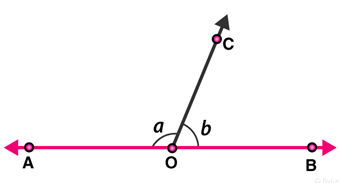Solution:

Given : ∠AOC and ∠BOC form a linear pair.

=> a + b = 1800 …..(1)

a – 2b = 300 …(2) (given)

On subtracting equation (2) from (1), we get

a + b – a + 2b = 180 – 30

3b = 150

b = 150/3

b = 500

Since, a – 2b = 300

a – 2(50) = 30

a = 30 + 100

a = 1300

Therefore, the values of a and b are 130° and 50° respectively.

Question 6: How many pairs of adjacent angles are formed when two lines intersect at a point?

Solution: Four pairs of adjacent angles are formed when two lines intersect each other at a single point.

For example, Let two lines AB and CD intersect at point O.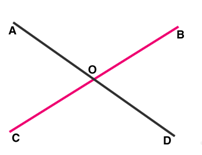The 4 pair of adjacent angles are :

(∠AOD,∠DOB),(∠DOB,∠BOC),(∠COA, ∠AOD) and (∠BOC,∠COA).

Question 7: How many pairs of adjacent angles, in all, can you name in figure given?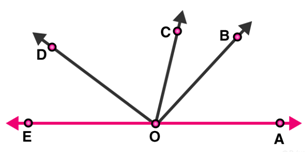Solution: Number of Pairs of adjacent angles, from the figure, are :

∠EOC and ∠DOC

∠EOD and ∠DOB

∠DOC and ∠COB

∠EOD and ∠DOA

∠DOC and ∠COA

∠BOC and ∠BOA

∠BOA and ∠BOD

∠BOA and ∠BOE

∠EOC and ∠COA

∠EOC and ∠COB

Hence, there are 10 pairs of adjacent angles.

Question 8: In figure, determine the value of x.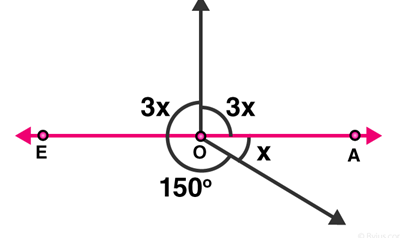Solution:

The sum of all the angles around a point O is equal to 360°.

Therefore,

3x + 3x + 150 + x = 3600

7x = 3600 – 1500

7x = 2100

x = 210/7

x = 300

Hence, the value of x is 30°.

Question 9: In figure, AOC is a line, find x.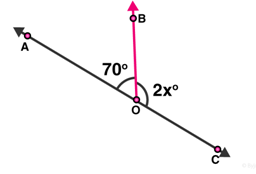Solution:

From the figure, ∠AOB and ∠BOC are linear pairs,

∠AOB +∠BOC =180°

70 + 2x = 180

2x = 180 – 70

2x = 110

x = 110/2

x = 55

Therefore, the value of x is 550.

Question 10: In figure, POS is a line, find x.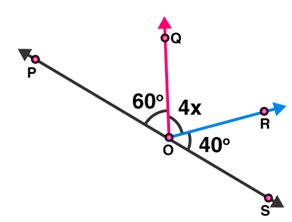Solution:

From figure, ∠POQ and ∠QOS are linear pairs.

Therefore,

∠POQ + ∠QOS=1800

∠POQ + ∠QOR+∠SOR=1800

600 + 4x +400 = 1800

4x = 1800 -1000

4x = 800

x = 200

Hence, the value of x is 200.

## RD Sharma Solutions for Class 9 Maths Chapter 8 Lines and Angles Exercise 8.2

Class 9 Maths Chapter 8 Lines and Angles Exercise 8.2 is based on topics such as some angle relations and linear pair of angles. Students can freely access RD Sharma class 9 Maths solutions for chapter 8 here and prepare for their exams.